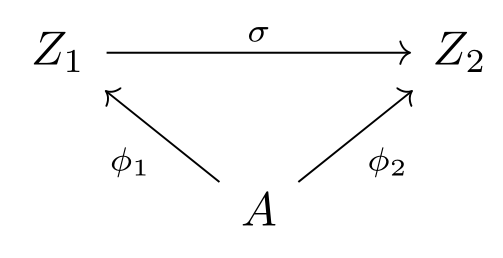Show Question
Math and science::Algebra::Aluffi

# Universal properties. Quotient.

A construction is said to satisfy a universal property or be the solution to a univeral problem when it may be viewed as a terminal object of a certain category.

The concept of a quotient can be viewed from this perspective. The idea is expressed (somewhat loosely) as follows:

### Quotients, as universal properties. Proposition.

Let $$\sim$$ be an equivalence relation defined on a set $$A$$.

The quotient $$A / \sim$$ is universal with respect to the property of mapping $$A$$ to a set in such a way that equivalent elements have the same image.

There is quite a lot that is implicit in this statement. A more explicit definition is as follows:

### Quotients, as initial objects. Proposition.

Let $$\sim$$ be an equivalence relation defined on a set $$A$$. Formulate a category $$\cat{C}$$ as follows:

• An object of $$\cat{C}$$ is any function $$\phi : A \to Z$$ to any set $$Z$$ such that for any $$a', a'' \in A, a' \sim a'' \implies \phi(a') = \phi(a'')$$.
• A morphism $$\sigma \in \cathom{C}(\phi_1, \phi_2)$$, for objects $$\phi_1 : A \to Z_1$$ and $$\phi_2 : A \to Z_2$$, is a function $$\sigma : Z_1 \to Z_2$$ such that $$\sigma \; \phi_1 = \phi_2$$.

Proposition: the function $$A \to A / \sim$$ is an initial object of the category $$\cat{C}$$.

The proof of this proposition is on the reverse side.

The flip side also has a diagram highlights the nature of the above category. It's a good exercise to try and guess it's form.

### Category diagram### Are there any final objects?

The category formulated above does have final objects, but they are not interesting: a function to a singleton set is a final object of the category.

### Essence

Any object in the category can be placed into a one-to-one correspondence with the quotient object and a morphism from the quotient. In other words, in the original set, any function $$f$$ satisfying the criteria (that elements of the same partition map to the same elements) can be uniquely decomposed into a composition of quotient function (function from set to partition) followed by a function $$g$$. In other words, there is a injection between all functions like $$f$$ and the functions like $$g$$ that compose with the quotient projection (not sure if projection is the right word here).

p33